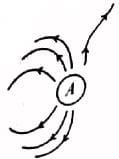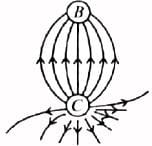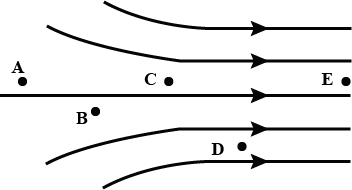NEET  >  Test: Electric Field Lines (NCERT)

# Test: Electric Field Lines (NCERT)

Test Description

## 10 Questions MCQ Test Physics Class 12 | Test: Electric Field Lines (NCERT)

Test: Electric Field Lines (NCERT) for NEET 2023 is part of Physics Class 12 preparation. The Test: Electric Field Lines (NCERT) questions and answers have been prepared according to the NEET exam syllabus.The Test: Electric Field Lines (NCERT) MCQs are made for NEET 2023 Exam. Find important definitions, questions, notes, meanings, examples, exercises, MCQs and online tests for Test: Electric Field Lines (NCERT) below.
Solutions of Test: Electric Field Lines (NCERT) questions in English are available as part of our Physics Class 12 for NEET & Test: Electric Field Lines (NCERT) solutions in Hindi for Physics Class 12 course. Download more important topics, notes, lectures and mock test series for NEET Exam by signing up for free. Attempt Test: Electric Field Lines (NCERT) | 10 questions in 10 minutes | Mock test for NEET preparation | Free important questions MCQ to study Physics Class 12 for NEET Exam | Download free PDF with solutions
 1 Crore+ students have signed up on EduRev. Have you?
Test: Electric Field Lines (NCERT) - Question 1

### Electric field lines provide information about

Test: Electric Field Lines (NCERT) - Question 2

### Which of the following figure represents the electric field lines due to a single positive charge?

Detailed Solution for Test: Electric Field Lines (NCERT) - Question 2

The field lines of a single positive charge are radially outward.

Test: Electric Field Lines (NCERT) - Question 3

### Which of the following figure represents the electric field lines due to a single negative charge?

Detailed Solution for Test: Electric Field Lines (NCERT) - Question 3

The field lines of single negative charge are radially inward.

Test: Electric Field Lines (NCERT) - Question 4

Which of the following figures correctly shows the top view sketch of the electric field lines for a uniformly charged hollow cylinder as shown in figure?Detailed Solution for Test: Electric Field Lines (NCERT) - Question 4

For a uniformly charged hollow cylinder, the electric field lines are as shown in figure (b).

Test: Electric Field Lines (NCERT) - Question 5

Figure shows the electric field lines around three point charges A, B and C. Which of the following charges are positive?Detailed Solution for Test: Electric Field Lines (NCERT) - Question 5

In the given figure, the electric lines of force emanate from A and C. Therefore, charges A and C must be positive.

Test: Electric Field Lines (NCERT) - Question 6

Figure shows the electric field lines around three point charges A, B and C. which charge has the largest magnitude?Detailed Solution for Test: Electric Field Lines (NCERT) - Question 6

From the given figure it is clear that, maximum number of electric lines of force are associated with charge C, so it must have the largest magnitude.

Test: Electric Field Lines (NCERT) - Question 7

Figure shows the electric field lines around three point charges A, B and C. which or regions of the figure could the electric field be zero?Detailed Solution for Test: Electric Field Lines (NCERT) - Question 7

From the figure, it is clear that, there cannot be a neutral point between a positive and a negative charge. Neutral point can be only there between two like charges i.e„ A and C. As magnitude of charge A is smaller than the magnitude of charge C, therefore, neutral point would lie closer to the charge A.

Test: Electric Field Lines (NCERT) - Question 8

A non-uniform electric field is represented by the diagram. At which of the following points the electric field is greatest in magnitude?Detailed Solution for Test: Electric Field Lines (NCERT) - Question 8

Electric field strength is maximum where the electric field lines are closer.

Test: Electric Field Lines (NCERT) - Question 9

Which of the following curves shown below cannot possibly represent electrostatic field lines?

Detailed Solution for Test: Electric Field Lines (NCERT) - Question 9

Electrostatic field lines start or end only at 900 to the surface of the conductor. So curve (b) cannot represent electrostatic field lines.

Test: Electric Field Lines (NCERT) - Question 10

Which of the following statements is not true about electric field lines?

Detailed Solution for Test: Electric Field Lines (NCERT) - Question 10

In a charge-free region, electric field lines can be taken to be continuous curves without any breaks.

## Physics Class 12

157 videos|452 docs|213 tests
 Use Code STAYHOME200 and get INR 200 additional OFF Use Coupon Code
Information about Test: Electric Field Lines (NCERT) Page
In this test you can find the Exam questions for Test: Electric Field Lines (NCERT) solved & explained in the simplest way possible. Besides giving Questions and answers for Test: Electric Field Lines (NCERT), EduRev gives you an ample number of Online tests for practice

## Physics Class 12

157 videos|452 docs|213 tests

### How to Prepare for NEET

Read our guide to prepare for NEET which is created by Toppers & the best Teachers